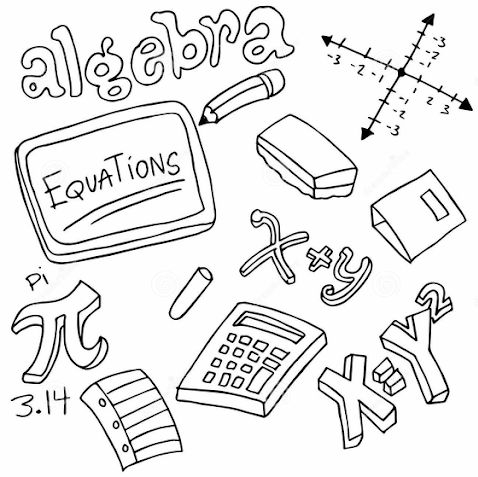# Simple Algebraic Equations

Welcome to Class !!

In today’s Mathematics class, We will be discussing Simple Algebraic Equations. We hope you enjoy the class!

CONTENT

• Solving equation by balance method
• Equation with bracket
• Equation with fractions.### Solving Equation by Balance Method

To solve an equation means to find the values of the unknown in the equation that makes it true.

For example: 2x – 9 = 15.

2x – 9 is on the left-hand side (LHS) and 15 is on the right-hand side (RHS) of the equals signs.

Worked examples

1. solve 3x = 12
2. 2x – 9 = 15.

Solution

1.3x = 12

Divide both sides by 3

1. 2x – 9 = 5

add 9 to both sides since +9 is the additive inverse of (-9)

2x – 9 + 9 = 15 + 9

2x = 24

x = 24/2  = 12

EVALUATION

Use the balance method to solve the following

(a) 3x – 8 = 10                      (b) 20 = 9x + 11       (c ) 10y – 7 = 27       (d) 9 + 2x = 16.

##### Equation with bracket

Worked example

1. solve 3(3x – 1) = 4  ( x + 3)
2. Solve 5 ( x + 11) + 2 ( 2x – 5)  = 0

Soluton

1. 3 (3x – 1) = 4 ( x + 3)

9x  – 3  = 4x + 12

Collect like terms:

9x – 4x = 12 + 3

5x = 15

x = 15/3

x  = 3

1. 5 (x + 11) + 2 ( 2x – 5)  = 0

5x + 55 + 4x – 10  = 0

collect like terms

5x + 5x = 10 – 55

9x  = -45

x = -45/9

x  = -5.

EVALUATION

Solve the following:

1. 2 (x + 5) = 18 2. 6 (2s – 7)  = 5s
2. 8 (2d – 3) = 3 (4d – 7) 5. (y + 8 ) + 2 (y + 1) = 0.
3. 3x + 1 = 2(3x+5)

#### Equation with Fraction

Before collecting like terms in an equation always clear the fraction. To clear fraction, multiply both sides of the equation by the L.C. M. of the denominators of the fraction.

Worked examples

Solve the equations.

Solution

EVALUATION

Solve the following equation

1.                              2.    x – 2     = 4

New General Mathematics chapt. 13 Ex 13d nos 1-20.

WEEKEND ASSIGNMENT

1. Solve 3x + 9 = 117

(a) 38             (b) 36    (c) -36  (d) -38

1. If -2r = 18 what is 4?

(a) -9              (b) 20             (c) 9   (d) -20

1. solve 2 (x + 5) = 16

(a) 13             (b) 10             (c)  8              (d) 3

1. Solve     (a) -15                (b) 15   (c) 10                       (d) -10
2. If = ½  What is x?     (a) 2 ½     (b) 2 2/3    (c) -2 ½   (d) 2.

THEORY

1. Solve the following   (a) 4 (x + 2) = 2 (3x – 1)    (b) 19y -2 (6y + 1)  = 8
2. Solve the following:

(a)

(b)

We have come to the end of this class. We do hope you enjoyed the class?

Should you have any further question, feel free to ask in the comment section below and trust us to respond as soon as possible.

We have come to the end of this term.  It’s been a remarkable journey and we are glad that you have made it this far. For making it this far, we commend you for being resilient, you have taken charge of your education and future.

The Journey still continues though, we are moving on to Second Term. we hope to meet you there.

Are you a Parent? Share your quick opinion and win free 2-month Premium Subscription

Don`t copy text!